# Definitions, simple applications, and graphs of trigonometric  (Page 6/7)

 Page 6 / 7
 $\theta$ 0 ${}^{\circ }$ 30 ${}^{\circ }$ 60 ${}^{\circ }$ 90 ${}^{\circ }$ 120 ${}^{\circ }$ 150 ${}^{\circ }$ $cos\theta$ $\theta$ 180 ${}^{\circ }$ 210 ${}^{\circ }$ 240 ${}^{\circ }$ 270 ${}^{\circ }$ 300 ${}^{\circ }$ 330 ${}^{\circ }$ 360 ${}^{\circ }$ $cos\theta$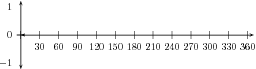Let us look back at our values for $cos\theta$

 $\theta$ ${0}^{\circ }$ ${30}^{\circ }$ ${45}^{\circ }$ ${60}^{\circ }$ ${90}^{\circ }$ ${180}^{\circ }$ $cos\theta$ 1 $\frac{\sqrt{3}}{2}$ $\frac{1}{\sqrt{2}}$ $\frac{1}{2}$ 0 $-1$

If you look carefully, you will notice that the cosine of an angle $\theta$ is the same as the sine of the angle ${90}^{\circ }-\theta$ . Take for example,

$cos{60}^{\circ }=\frac{1}{2}=sin{30}^{\circ }=sin\left({90}^{\circ }-{60}^{\circ }\right)$

This tells us that in order to create the cosine graph, all we need to do is to shift the sine graph ${90}^{\circ }$ to the left. The graph of $cos\theta$ is shown in [link] . As the cosine graph is simply a shifted sine graph, it will have the same period and amplitude as the sine graph.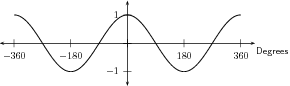The graph of cos θ .

## Functions of the form $y=acos\left(x\right)+q$

In the equation, $y=acos\left(x\right)+q$ , $a$ and $q$ are constants and have different effects on the graph of the function. The general shape of the graph of functions of this form is shown in [link] for the function $f\left(\theta \right)=2cos\theta +3$ .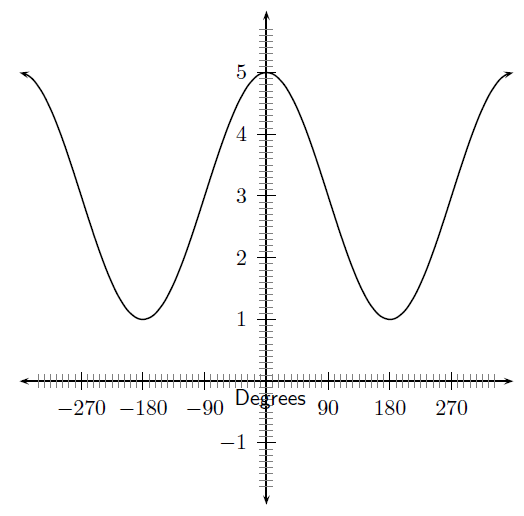Graph of f ( θ ) = 2 cos θ + 3

## Functions of the form $y=acos\left(\theta \right)+q$ :

1. On the same set of axes, plot the following graphs:
1. $a\left(\theta \right)=cos\theta -2$
2. $b\left(\theta \right)=cos\theta -1$
3. $c\left(\theta \right)=cos\theta$
4. $d\left(\theta \right)=cos\theta +1$
5. $e\left(\theta \right)=cos\theta +2$
Use your results to deduce the effect of $q$ .
2. On the same set of axes, plot the following graphs:
1. $f\left(\theta \right)=-2·cos\theta$
2. $g\left(\theta \right)=-1·cos\theta$
3. $h\left(\theta \right)=0·cos\theta$
4. $j\left(\theta \right)=1·cos\theta$
5. $k\left(\theta \right)=2·cos\theta$
Use your results to deduce the effect of $a$ .

You should have found that the value of $a$ affects the amplitude of the cosine graph in the same way it did for the sine graph.

You should have also found that the value of $q$ shifts the cosine graph in the same way as it did the sine graph.

These different properties are summarised in [link] .

 $a>0$ $a<0$ $q>0$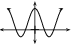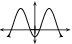$q<0$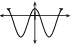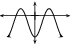## Domain and range

For $f\left(\theta \right)=acos\left(\theta \right)+q$ , the domain is $\left\{\theta :\theta \in \mathbb{R}\right\}$ because there is no value of $\theta \in \mathbb{R}$ for which $f\left(\theta \right)$ is undefined.

It is easy to see that the range of $f\left(\theta \right)$ will be the same as the range of $asin\left(\theta \right)+q$ . This is because the maximum and minimum values of $acos\left(\theta \right)+q$ will be the same as the maximum and minimum values of $asin\left(\theta \right)+q$ .

## Intercepts

The $y$ -intercept of $f\left(\theta \right)=acos\left(x\right)+q$ is calculated in the same way as for sine.

$\begin{array}{ccc}\hfill {y}_{int}& =& f\left({0}^{\circ }\right)\hfill \\ & =& acos\left({0}^{\circ }\right)+q\hfill \\ & =& a\left(1\right)+q\hfill \\ & =& a+q\hfill \end{array}$

## Comparison of graphs of $sin\theta$ And $cos\theta$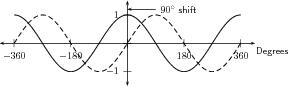The graph of cos θ (solid-line) and the graph of sin θ (dashed-line).

Notice that the two graphs look very similar. Both oscillate up and down around the $x$ -axis as you move along the axis. The distances between the peaks of the two graphs is the same and is constant along each graph. The height of the peaks and the depths of the troughs are the same.

The only difference is that the $sin$ graph is shifted a little to the right of the $cos$ graph by 90 ${}^{\circ }$ . That means that if you shift the whole $cos$ graph to the right by 90 ${}^{\circ }$ it will overlap perfectly with the $sin$ graph. You could also move the $sin$ graph by 90 ${}^{\circ }$ to the left and it would overlap perfectly with the $cos$ graph. This means that:

$\begin{array}{ccc}\hfill sin\theta & =& cos\left(\theta -90\right)\phantom{\rule{1.em}{0ex}}\left(\mathrm{shift}\phantom{\rule{2.pt}{0ex}}\mathrm{the}cos\mathrm{graph}\phantom{\rule{2.pt}{0ex}}\mathrm{to}\phantom{\rule{2.pt}{0ex}}\mathrm{the}\phantom{\rule{2.pt}{0ex}}\mathrm{right}\right)\hfill \\ & \mathbf{a}\mathrm{nd}& \\ \hfill cos\theta & =& sin\left(\theta +90\right)\phantom{\rule{1.em}{0ex}}\left(\mathrm{shift}\phantom{\rule{2.pt}{0ex}}\mathrm{the}sin\mathrm{graph}\phantom{\rule{2.pt}{0ex}}\mathrm{to}\phantom{\rule{2.pt}{0ex}}\mathrm{the}\phantom{\rule{2.pt}{0ex}}\mathrm{left}\right)\hfill \end{array}$

## Graph of $tan\theta$

Complete the following table, using your calculator to calculate the values correct to 1 decimal place. Then plot the values with $tan\theta$ on the $y$ -axis and $\theta$ on the $x$ -axis.

 $\theta$ 0 ${}^{\circ }$ 30 ${}^{\circ }$ 60 ${}^{\circ }$ 90 ${}^{\circ }$ 120 ${}^{\circ }$ 150 ${}^{\circ }$ $tan\theta$ $\theta$ 180 ${}^{\circ }$ 210 ${}^{\circ }$ 240 ${}^{\circ }$ 270 ${}^{\circ }$ 300 ${}^{\circ }$ 330 ${}^{\circ }$ 360 ${}^{\circ }$ $tan\theta$#### Questions & Answers

Is there any normative that regulates the use of silver nanoparticles?
Damian Reply
what king of growth are you checking .?
Renato
What fields keep nano created devices from performing or assimulating ? Magnetic fields ? Are do they assimilate ?
Stoney Reply
why we need to study biomolecules, molecular biology in nanotechnology?
Adin Reply
?
Kyle
yes I'm doing my masters in nanotechnology, we are being studying all these domains as well..
Adin
why?
Adin
what school?
Kyle
biomolecules are e building blocks of every organics and inorganic materials.
Joe
anyone know any internet site where one can find nanotechnology papers?
Damian Reply
research.net
kanaga
sciencedirect big data base
Ernesto
Introduction about quantum dots in nanotechnology
Praveena Reply
what does nano mean?
Anassong Reply
nano basically means 10^(-9). nanometer is a unit to measure length.
Bharti
do you think it's worthwhile in the long term to study the effects and possibilities of nanotechnology on viral treatment?
Damian Reply
absolutely yes
Daniel
how to know photocatalytic properties of tio2 nanoparticles...what to do now
Akash Reply
it is a goid question and i want to know the answer as well
Maciej
characteristics of micro business
Abigail
for teaching engĺish at school how nano technology help us
Anassong
Do somebody tell me a best nano engineering book for beginners?
s. Reply
there is no specific books for beginners but there is book called principle of nanotechnology
NANO
what is fullerene does it is used to make bukky balls
Devang Reply
are you nano engineer ?
s.
fullerene is a bucky ball aka Carbon 60 molecule. It was name by the architect Fuller. He design the geodesic dome. it resembles a soccer ball.
Tarell
what is the actual application of fullerenes nowadays?
Damian
That is a great question Damian. best way to answer that question is to Google it. there are hundreds of applications for buck minister fullerenes, from medical to aerospace. you can also find plenty of research papers that will give you great detail on the potential applications of fullerenes.
Tarell
what is the Synthesis, properties,and applications of carbon nano chemistry
Abhijith Reply
Mostly, they use nano carbon for electronics and for materials to be strengthened.
Virgil
is Bucky paper clear?
CYNTHIA
carbon nanotubes has various application in fuel cells membrane, current research on cancer drug,and in electronics MEMS and NEMS etc
NANO
so some one know about replacing silicon atom with phosphorous in semiconductors device?
s. Reply
Yeah, it is a pain to say the least. You basically have to heat the substarte up to around 1000 degrees celcius then pass phosphene gas over top of it, which is explosive and toxic by the way, under very low pressure.
Harper
Do you know which machine is used to that process?
s.
how to fabricate graphene ink ?
SUYASH Reply
for screen printed electrodes ?
SUYASH
What is lattice structure?
s. Reply
of graphene you mean?
Ebrahim
or in general
Ebrahim
in general
s.
Graphene has a hexagonal structure
tahir
On having this app for quite a bit time, Haven't realised there's a chat room in it.
Cied
what is biological synthesis of nanoparticles
Sanket Reply
Got questions? Join the online conversation and get instant answers!
Jobilize.com Reply

### Read also:

#### Get the best Algebra and trigonometry course in your pocket!

Source:  OpenStax, Math 1508 (lecture) readings in precalculus. OpenStax CNX. Aug 24, 2011 Download for free at http://cnx.org/content/col11354/1.1
Google Play and the Google Play logo are trademarks of Google Inc.

Notification Switch

Would you like to follow the 'Math 1508 (lecture) readings in precalculus' conversation and receive update notifications?By Mistry BhaveshBy